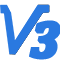从牛顿大炮说起，谈谈火箭入轨的控制原理

一、从牛顿大炮说起

牛顿曾说在一座高山上架起一门大炮，只要这门炮的威力足够大，炮弹的速度足够快，炮弹就可以围绕地球不停的转而不会掉下来。那么对于一枚火箭，速度多快才能入轨？中学课本告诉我们，只要达到第一宇宙速度7.9km/s，我们的火箭就能够进入地球轨道。

以上便是大家熟知的火箭入轨的方法。但是请思考以下问题：

(1) 速度大小必须达到7.9千米每秒吗？

(2) 对速度方向没有要求吗？

(3) 轨道的形状怎么确定？

(4) 怎么控制火箭进入预定的轨道?

牛顿大炮的例子中，我们可以感觉到以上问题的答案。大炮在山顶的位置、炮弹的出膛速度、以及速度的角度，共同决定了炮弹的轨道的形状。下面我们就来谈谈这些问题。

二、火箭入轨的过程

火箭入轨可以简单分为以下几个过程。

(1) 垂直起飞。发动机点火，待发动机推力稳定后，放开火箭。火箭离开发射塔，快速穿过稠密的底层大气层。

(2) 程序转弯。启动转弯过程，以适当的角度穿越跨声速段，防止箭体结构破坏。同时使得火箭在关机点达到所需的速度方向。

(3) 主动段关机。保持速度方向，不断加速，当速度达到所需速度时，执行发动机关机指令。

(4) 星箭分离。分离卫星和火箭，启动反推火箭，让火箭刹车，以免碰撞卫星。同时使火箭减速落回地面。

(5) 入轨。卫星进入轨道。

其中（1）（2）（3）并称为主动段，（4）（5）称为被动段。火箭入轨后的参数唯一取决于主动段关机点的参数。即火箭从发射架上起飞，飞到某个位置然后执行发动机关机指令。在关机点的位置、速度、速度方向就唯一确定了其入轨后的轨道参数。

如图3个关机点参数为：到地心的距离$r_k$、速度大小$V_k$、弹道倾角$\Theta _{k}$。那么如何根据想要的卫星的轨道参数，确定所需要的主动段关机点参数呢?

[修改于 10 个月前 - 2019-07-27 03:44:00]

来自：航天航空 / 喷气推进航天航空 / 空间技术

3
2019-7-27 2:57:55忆昔长别（作者）
1楼

三、由轨道参数计算关机点参数

中学物理就讲过，卫星的轨道形状是一个椭圆。地球在椭圆的一个焦点上，而椭圆的参数包括半长轴a、偏心率e。

在研究轨道问题时，常常在极坐标系下进行，如下图所示。

图中，$p$为近地点，$a$为远地点。已知轨道近地点到地心的距离$r_a$，远地点到地心的距离$r_p$，近地点的位置$p$，关机点与地心连线与$op$的夹角为$f_k$。那么关机点参数可以用下面的公式进行计算。

关机点到地心的距离

$$r_k=\frac{p}{1+e\cdot cosf}$$

关机点的速度大小

$$V_k=\sqrt{\frac{\mu }{p}(1+2\cdot e \cdot cosf+e^2))}$$

关机点弹道倾角为（弹道倾角：速度方向与当地水平面的夹角）

$$tan\Theta =\frac{e\cdot sinf}{1+e\cdot cosf}$$

其中，

$\mu$为地球引力常数，$\mu=3.986\times 10^{14}$，

$e$为椭圆轨道偏心率,$e=\frac{r_a-r_p}{r_a+r_p}$,

$a$为椭圆半长轴,$a=\frac{r_a+r_p}{2}$,

$p$为椭圆半通径，$p=a\cdot (1-e^2)$。

此外要想成为地球卫星，还有一些附加条件。

（1）关机点速度应小于第二宇宙速度，因为大于第二宇宙速度的话就飞离地球了。

（2）弹道倾角应在0~90（不包括90度）度之间，$\Theta=90^{\circ}$垂直向上发射火箭一定会落下来。

（3）椭圆轨道不应与地面有交点。因为砸到人不好。

[修改于 10 个月前 - 2019-07-27 03:38:45]

加载评论中，请稍候...
2019-7-27 3:12:01忆昔长别（作者）
2楼

四、举例

打算发射一枚火箭入轨，轨道参数是这样的。在近地点到地面的高度为100km，远地点到地面的高度为200km，关机点和近地点的夹角是120度。已知地球平均半径R=6371km，问火箭主动段关机点的参数？

解：,远地点到地心距离

$$r_a=6371+200=6571km$$

近地点到地心距离

$$r_p=6371+100=6471km$$

偏心率

$$e=\frac{r_a-r_p}{r_a+r_p}=0.00767$$

半长轴

$$a=\frac{r_a+r_p}{2}=6531km$$

半通径

$$p=a\cdot (1-e^2)=6520.62km$$

关机点到地心的距离

$$r_k=\frac{p}{1+e\cdot cosf}=6545.72km$$

关机点的海拔高度为

$$h_k=r_k-R=174.72km$$

关机点的速度大小

$$V_k=\sqrt{\frac{\mu }{p}(1+2\cdot e \cdot cosf+e^2))}=7.79km/s$$

关机点弹道倾角为（弹道倾角：速度方向与当地水平面的夹角）

$$tan\Theta =\frac{e\cdot sinf}{1+e\cdot cosf}=6.67/time 10^{-3}，\Theta=0.38^{\circ}$$

所以想要获得所需轨道，只需在距地面174.72千米处，使得关机点的速度为7.79千米每秒，速度与当地水平面的夹角为0.38度即可。

可能有人会奇怪，为什么关机点速度小于7.9km/s？这是因为7.9km/s是第一宇宙速度，也是成为人造卫星的最大速度，即人造卫星贴地飞行时速度是7.9m/s。事实上卫星距离地面越远，则速度越慢。举个极端例子，近地轨道卫星一天能绕地球十几圈，而同步轨道上的卫星几乎相对地面静止。

五、小结

(1)火箭入轨后的轨道唯一取决于主动段关机点的参数。

(2)可以根据椭圆轨道的参数计算出关机点的参数。

(3)通过设计火箭主动段弹道，即可实现火箭进入预定轨道。

(4)控制的过程是，（a）首先火箭垂直起飞，以最快速度离开稠密的底层大气，减少能量消耗。（b）然后进行程序转弯，在跨声速段，保持攻角为零，避免火箭受气动力过大导致结构破坏，最后要转弯到指定的弹道倾角，并保持这个角度。（c）当火箭加速到关机速度时，执行关机指令，发动机关机。启动反推火箭，星箭分离，卫星进入轨道。

(5)注意设计的椭圆轨道不应与地面有交点，会变成弹道导弹的，会砸到人的

END

[修改于 10 个月前 - 2019-07-27 03:34:56]

加载评论中，请稍候...
3楼

一般来说...如果不是为了进入转移轨道都不会搞偏心率太大的椭圆轨道...

嗯，轨道不与地面相交原因只是因为砸到人...而且应该加一句近地点最好也不要在太低的位置，除非入轨之后立即抬升或者不再经过近地点。

加载评论中，请稍候...忆昔长别（作者）
4楼

算的时候发现了，偏心率真是相当接近0，几乎是圆形轨道了。

我记得高纬度地区的通信卫星是大椭圆轨道，远地点运行很慢，近地点很快，可以保证星下点大部分时间都在高纬度地区。

远程火箭一级二级落点也是可以计算的，算好落点以免落到有人区，砸到人。

加载评论中，请稍候...Robot_V15楼

玩过ksp就很容易理解了

加载评论中，请稍候...3141526楼
引用Robot_V1发表于5楼的内容
玩过ksp就很容易理解了

加载评论中，请稍候...忆昔长别（作者）
7楼
引用Robot_V1发表于5楼的内容
玩过ksp就很容易理解了

感觉坎巴拉就是低配版本的stk。玩这样一个游戏也是相当不错的。

加载评论中，请稍候...
2019-08-06 11:34:46
2019-8-6 11:34:46dkhtt8楼

厉害，都开始计算轨道了

加载评论中，请稍候...
2020-05-15 19:28:09
2020-5-15 19:28:09
9楼

记得当时我都是自学这些的推荐有个叫航天模拟器的手游，适宜作为ksp的入门版（二维的），玩这个就容易懂了。

加载评论中，请稍候...

想参与大家的讨论？现在就 登录 或者 注册忆昔长别进士 学者 机友 笔友
文章
7
回复
66
学术分
1
2015/12/24注册，2 个月前活动
暂无简介
作者最新文章
相似文章推荐
插入资源
全部
图片
视频
音频
附件
全部
未使用
已使用
正在上传
空空如也~
上传中..{{f.progress}}%
处理中..
上传失败，点击重试
{{f.name}}
空空如也~
(视频){{r.oname}}
{{selectedResourcesId.indexOf(r.rid) + 1}}
插入表情
我的表情
共享表情
Emoji
上传
##### 注意事项
最大尺寸100px，超过会被压缩。为保证效果，建议上传前自行处理。
建议上传自己DIY的表情，严禁上传侵权内容。
点击重试等待上传{{s.progress}}%处理中...已上传
空空如也~
草稿箱
加载中...
此处只插入正文，如果要使用草稿中的其余内容，请点击继续创作。
{{fromNow(d.toc)}}
{{getDraftInfo(d)}}
标题：{{d.t}}
内容：{{d.c}}
{{forum.displayName}}